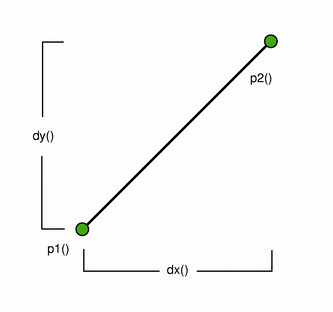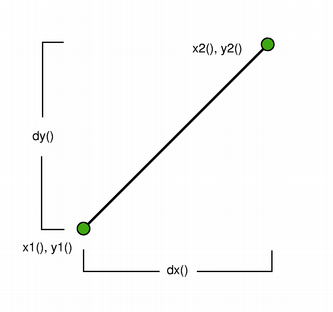# QLine Class

The QLine class provides a two-dimensional vector using integer precision. More...

 Header: #include qmake: QT += core

## Public Functions

 QLine() QLine(const QPoint &p1, const QPoint &p2) QLine(int x1, int y1, int x2, int y2) QPoint p1() const QPoint p2() const int x1() const int x2() const int y1() const int y2() const QPoint center() const int dx() const int dy() const bool isNull() const void setP1(const QPoint &p1) void setP2(const QPoint &p2) void setLine(int x1, int y1, int x2, int y2) void setPoints(const QPoint &p1, const QPoint &p2) void translate(const QPoint &offset) void translate(int dx, int dy) QLine translated(const QPoint &offset) const QLine translated(int dx, int dy) const bool operator!=(const QLine &line) const bool operator==(const QLine &line) const
 QDataStream & operator<<(QDataStream &stream, const QLine &line) QDataStream & operator>>(QDataStream &stream, QLine &line)

## Detailed Description

The QLine class provides a two-dimensional vector using integer precision.

A QLine describes a finite length line (or a line segment) on a two-dimensional surface. The start and end points of the line are specified using integer point accuracy for coordinates. Use the QLineF constructor to retrieve a floating point copy.The positions of the line's start and end points can be retrieved using the p1(), x1(), y1(), p2(), x2(), and y2() functions. The dx() and dy() functions return the horizontal and vertical components of the line. Use isNull() to determine whether the QLine represents a valid line or a null line.

Finally, the line can be translated a given offset using the translate() function.

See also QLineF, QPolygon, and QRect.

## Member Function Documentation

### QLine::QLine()

Constructs a null line.

### QLine::QLine(const QPoint &p1, const QPoint &p2)

Constructs a line object that represents the line between p1 and p2.

### QLine::QLine(intx1, inty1, intx2, inty2)

Constructs a line object that represents the line between (x1, y1) and (x2, y2).

### QPoint QLine::p1() const

Returns the line's start point.

See also setP1(), x1(), y1(), and p2().

### QPoint QLine::p2() const

Returns the line's end point.

See also setP2(), x2(), y2(), and p1().

### int QLine::x1() const

Returns the x-coordinate of the line's start point.

See also p1().

### int QLine::x2() const

Returns the x-coordinate of the line's end point.

See also p2().

### int QLine::y1() const

Returns the y-coordinate of the line's start point.

See also p1().

### int QLine::y2() const

Returns the y-coordinate of the line's end point.

See also p2().

### QPoint QLine::center() const

Returns the center point of this line. This is equivalent to (p1() + p2()) / 2, except it will never overflow.

This function was introduced in Qt 5.8.

### int QLine::dx() const

Returns the horizontal component of the line's vector.

See also dy().

### int QLine::dy() const

Returns the vertical component of the line's vector.

See also dx().

### bool QLine::isNull() const

Returns `true` if the line is not set up with valid start and end point; otherwise returns `false`.

### void QLine::setP1(const QPoint &p1)

Sets the starting point of this line to p1.

This function was introduced in Qt 4.4.

See also setP2() and p1().

### void QLine::setP2(const QPoint &p2)

Sets the end point of this line to p2.

This function was introduced in Qt 4.4.

See also setP1() and p2().

### void QLine::setLine(intx1, inty1, intx2, inty2)

Sets this line to the start in x1, y1 and end in x2, y2.

This function was introduced in Qt 4.4.

See also setP1(), setP2(), p1(), and p2().

### void QLine::setPoints(const QPoint &p1, const QPoint &p2)

Sets the start point of this line to p1 and the end point of this line to p2.

This function was introduced in Qt 4.4.

See also setP1(), setP2(), p1(), and p2().

### void QLine::translate(const QPoint &offset)

Translates this line by the given offset.

### void QLine::translate(intdx, intdy)

This is an overloaded function.

Translates this line the distance specified by dx and dy.

### QLine QLine::translated(const QPoint &offset) const

Returns this line translated by the given offset.

This function was introduced in Qt 4.4.

### QLine QLine::translated(intdx, intdy) const

This is an overloaded function.

Returns this line translated the distance specified by dx and dy.

This function was introduced in Qt 4.4.

### bool QLine::operator!=(const QLine &line) const

Returns `true` if the given line is not the same as this line.

A line is different from another line if any of their start or end points differ, or the internal order of the points is different.

### bool QLine::operator==(const QLine &line) const

Returns `true` if the given line is the same as this line.

A line is identical to another line if the start and end points are identical, and the internal order of the points is the same.

## Related Non-Members

### QDataStream &operator<<(QDataStream &stream, const QLine &line)

Writes the given line to the given stream and returns a reference to the stream.

See also Serializing Qt Data Types.

### QDataStream &operator>>(QDataStream &stream, QLine &line)

Reads a line from the given stream into the given line and returns a reference to the stream.

See also Serializing Qt Data Types.

© 2019 The Qt Company Ltd. Documentation contributions included herein are the copyrights of their respective owners. The documentation provided herein is licensed under the terms of the GNU Free Documentation License version 1.3 as published by the Free Software Foundation. Qt and respective logos are trademarks of The Qt Company Ltd. in Finland and/or other countries worldwide. All other trademarks are property of their respective owners.